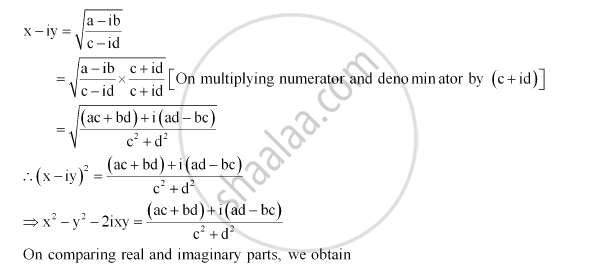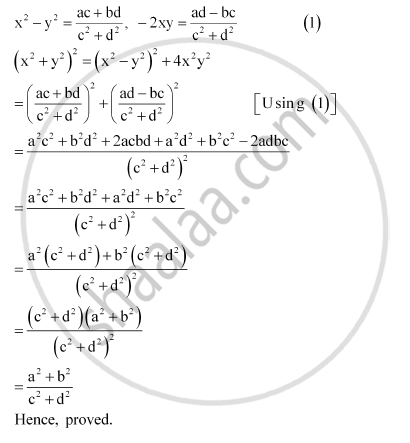CBSE (Arts) Class 11CBSE
Share

# If X – Iy = Sqrt((A-ib)/(C - Id)) Prove that (Xsquare2 + Ysquare2) = (Asquare2 + Bsquare2)/(Csquare2 + Dsquare2) - CBSE (Arts) Class 11 - Mathematics

ConceptAlgebra of Complex Numbers

#### Question

If x – iy = sqrt((a-ib)/(c - id)) prove that (x^2 + y^2) = (a^2 + b^2)/(c^2 + d^2)

#### SolutionIs there an error in this question or solution?

#### Video TutorialsVIEW ALL 

Solution If X – Iy = Sqrt((A-ib)/(C - Id)) Prove that (Xsquare2 + Ysquare2) = (Asquare2 + Bsquare2)/(Csquare2 + Dsquare2) Concept: Algebra of Complex Numbers.
S